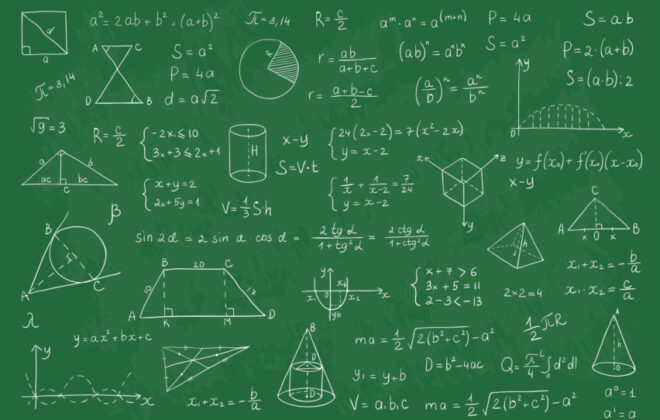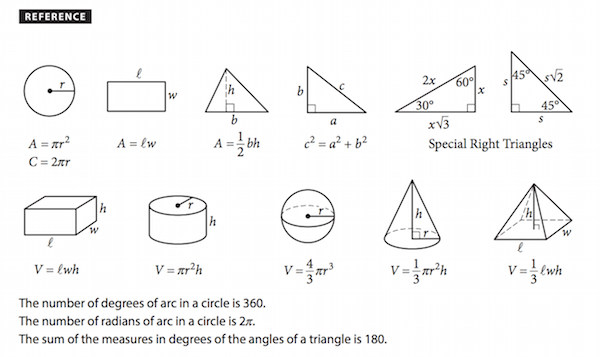# The 30 Most Important SAT & ACT Math Formulas to Learn

April 11, 2023Does the very word “math” strike fear into your heart? If so, you’re not alone. But if you’re applying to college and taking the SAT or ACT, you will need to know some critical math formulas. Knowing these formulas won’t only keep you from wasting time on test day referencing the formula sheet, they’ll also improve your confidence so that you can go in there and tackle that test! In this guide, we cover the most important SAT formulas and ACT math formulas to know, as well as the basic formats of each standardized test and how they differ. And if you’re looking for more information on which high school math class is right for you before the day of that big test, check out our guide here.

## Format of the ACT Math Section

Let’s get into the nitty-gritty! The mathematics section of the ACT, which follows the English section, includes 60 multiple-choice questions, with five possible answers for each. You’ll have 60 minutes to complete this section, which comes out to approximately 1 minute per question. Approved calculators are permitted for the entire math section of the ACT. The ACT questions progress in order of difficulty, starting with the easiest and ending with the hardest.

Note that the ACT does not provide a formula sheet. This is why it is essential that you review our guide below on the most important ACT math formulas.

## What is Included in the ACT Math Section?

The ACT Math section breaks down into three categories. The first is Preparing for Higher Math, which comprises 35 questions and accounts for approximately 60% of your math score. The Preparing for Higher Math section breaks down into the following subsections:

1. Number & Quantity: 7-10%
2. Algebra: 12-15%
3. Functions: 12-15%
4. Geometry: 12-15%
5. Statistics & Probability: 8-12%

The second category is Integrating Essential Skills, which is 25 questions and approximately 40% of your math score. The third category is Modeling, which is found in the two categories above, and asks you to draw diagrams or models of some of the problems. Since each of the subsections in the Preparing for Higher Math portion of the exam receives its own score, you will receive eight reporting category scores for the math section, as well as your total math score between 1-36.

Since the ACT requires a working knowledge of Geometry and Algebra II, you should wait until the late spring of your junior year to take the test. However, if you’re an advanced math student already taking these courses, you can opt for an earlier test date. For more information on how to choose when to sit for the ACT, check out this helpful article.

## Format of the SAT Math Section

There are two sections to the mathematics component of the SAT. One of these sections is math with a calculator, and the other is without a calculator. Unlike the ACT, which only has multiple-choice questions, the SAT has multiple-choice and “grid-in” free-form questions. Additionally, you will be given an SAT formula sheet for the math section, which you can find here.

The SAT math section has 54 questions, which test-takers have 70 minutes to complete. Some of these questions have multiple parts and thus rely on numerous different math formulas.

## What is Included in the SAT Math Section?

The SAT measures students’ knowledge and aptitude in three significant areas, which are defined as follows:

• Heart of Algebra (19 questions): Linear equations, systems of linear equations, and functions. This section tests your ability to apply algebraic concepts and their relationships to real-world applications.
• Problem Solving and Data Analysis (17 questions): Ratios, proportions, percentages, analysis of graphs, quantitative reasoning, and data interpretation skills. You will likely encounter charts, graphs, and tables in this section.
• Passport to Advanced Math (16 questions): This section focuses on more advanced math concepts, such as quadratic and higher-order equations, manipulating polynomials, and more complex equations or functions.
• Additional Topics (6 questions): Geometric concepts, fundamentals of trigonometry, and the arithmetic of complex numbers.

Like the ACT, the SAT involves high-level math concepts in algebra and geometry that many students study in the latter half of junior year. For more information on how to choose when to take the SAT, refer to this guide.

## SAT vs. ACT: Which is Right for Me?

Though the SAT and ACT math sections share much in common and draw from the same essential skillset, they each test different skills. The SAT requires more critical thinking and reasoning skills, while the ACT requires more rote memorization—and thus, more formulas to memorize. When in doubt about which to take, we recommend doing a practice test of each and seeing where your natural abilities shine. And if you don’t score well the first time you take the test, don’t worry! Studies show that consistent practice improves students’ scores by one hundred points or more.

## How to Study for the SAT/ACT Math Sections

If you’re a high school student planning to take the SAT or ACT, you may be wondering how to best prepare for the math portion of the test. The good news is that you’ve likely been preparing for years, as both these tests are designed to draw on the material you’ve covered in school. Practice, however, makes perfect.

To prepare for the SAT and ACT, take practice tests. These tests are the best way to familiarize yourself with the types of questions you’ll be asked on the exam. As you practice, score yourself to find your weaknesses. Use these weaknesses as a guide to direct your studying.

Additionally, memorize the key formulas below. Knowing these important ACT and SAT formulas can help you save time on questions. Some memorization tips include making flashcards, drawing visual aids such as diagrams and charts, and using mnemonics.

Finally, many online resources and tutoring services are available for SAT/ACT math prep. Khan Academy, for instance, offers free SAT prep courses. Just 20 hours of Khan Academy practice can lead to an increase of over 100 points on your SAT.

## Algebra & Coordinate Geometry Formulas to Know

#### 1) Arithmetic mean (average) = (sum of all numbers) / (number of numbers)

For example, if you have the number set {2, 4, 6, 8, 10}, you can find the arithmetic mean as follows: {2 + 4 + 6 + 8 + 10}/5 = 30/5 = 6

#### 2) Quadratic equation: x = −b ± √b²-4ac/2a

Used to solve quadratic equations of ax^2 + bx + c = 0. Plug in the values of a, b, and c and solve for x.

#### 3) Slope Formula: (y₂-y₁)/ (x₂– x₁)

The slope is the rise (vertical change) / run (horizontal change)

#### 4) Distance Formula: d=√(x₁ – x₂)² + (y₁ – y₂)²

Calculates the distance between two points on a coordinate plane.

#### 5) Slope-intercept form of a line: y= mx+b

Describes the equation of a line in terms of its slope (m) and y-intercept.

#### 6) Midpoint formula: (x₁+x₂) / 2, (y₁+y₂) / 2

Calculates the midpoint of a line segment on a coordinate plane.

#### 7) Point-slope form of a line: y – y1 = m(x – x1)

Equation of a straight line, where m is the slope and (x1, y1) is a point on the line.

## Plane Geometry Formulas to Know

Note that you’ll be given the following reference sheet for the SAT. While memorizing these important math formulas is helpful to save time, you will see them on test day if you’re taking the SAT.9) Area of triangle: area = (1/2) (base) (height)

10) Pythagorean theorem: a²+b²=c²

11) Special types of triangles: 45-45-90 triangles and 30-60-90 triangles

12) Area of a circle: π * r²

13) Area of a sector in a circle: 𝜃/360o(𝜋r2)

14) Area of a rectangle: area = length x width

15) Area of a parallelogram: area = base x height

16) Area of a trapezoid: A = ½ (a + b) h

17) Circumference of a Circle: C = 2πr

18) Sum of interior angles of a polygon: ( n − 2 ) × 180, where n= number of sides

19) Surface area of a sphere: 4πr2

Sequence Formulas to Know:

20) Arithmetic Sequence: an=a1 + c(n – 1)

21) Geometric Sequence: an=a1( c)n – 1

## Trigonometry Formulas to Know

Remember: SOHCAHTOA

22) Sine = opposite/hypotenuse

## Statistics & Probability Formulas to Know

25) Probability = Number of favorable outcomes / Total number of outcomes

26) Simple interest formula: Principal x Rate x Time

27) Compound interest formula: Final amount = Principal x (1 + Rate/n)^(n x Time)

28) Percentages: percent = part / whole x 100%

Percent change =. change / original

29) Permutations: nPr = n! / (n-r)!, where n is the total number of objects and r is the number of objects being selected

30) Combinations: nCr = n! / (r! * (n-r)!), where n is the total number of objects and r is the number of objects being selected

## In Conclusion – The Most Important SAT/ACT Math Formulas to Know

For many students, taking the SAT and ACT is an essential rite of passage. However, these tests can be stressful, so the more prepared you are, the better. Remember to study hard, take practice tests, and memorize the important math formulas above. The night before the big exam, get plenty of rest so that you wake up the following day ready to go. During the exam, keep an eye on the time. If you get stuck, try to eliminate whatever answers you can to help you make an educated guess. Lastly, remember to check your work before you hand it in. With proper preparation, you’ll be well on your way to success!